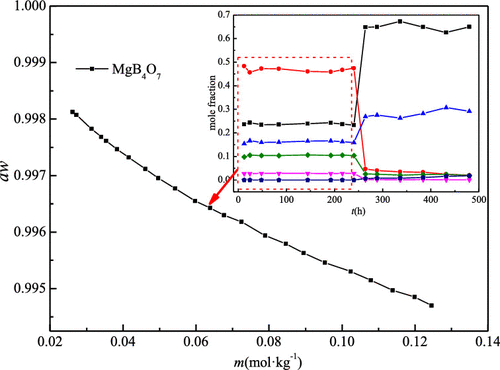Journal of Chemical & Engineering Data ( IF 2.369 ) Pub Date : 2019-12-23 , DOI: 10.1021/acs.jced.9b00663
Haiwen Ge; Min Wang; Yan Yao; Tianlong DengThe solubility and behavior of phase transformation for MgB4O7 + H2O were studied at 298.15 K by the method of isothermal dissolution equilibrium. The results indicated that the equilibrium solid phase MgB4O7·9H2O was kept in the aqueous solution for 240 h and started to transform into inderite (MgB3O3(OH)5·5H2O) after 240 h. The concentration of the equilibrium liquid phase with respect to MgB4O7·9H2O is 0.03494 mol·kg–1 as metastable solubility. The isopiestic solubility, the osmotic coefficient, and water activity for the saturated solution of MgB4O7·9H2O at 298.15 ± 0.01 K were determined by the isopiestic method first, and obtained the values of 0.03536 mol·kg–1, 1.8753, and 0.99761, respectively. The Pitzer’s ion-interaction parameters and the equilibrium constant ln K for the MgB4O7 + H2O system at 298.15 K were obtained by fitting the experimental data of osmotic coefficients and the measurements of solubility and activity properties by the isopiestic method using the Pitzer equation of the osmotic coefficient with an adjusted α2 and the equilibrium constant equation simultaneously. The standard deviation is 0.005391 for the fit. The solubility of MgB4O7·9H2O was calculated using the parameterized equations and the lnK of 0.71826. The calculated isopiestic solubility is 0.03530 mol·kg–1, which agrees well with isothermal solubility and experimental isopiestic solubility.

down
wechat
bug